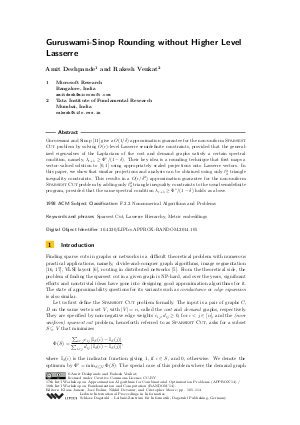Document# Guruswami-Sinop Rounding without Higher Level Lasserre

### Authors Amit Deshpande, Rakesh Venkat## File

LIPIcs.APPROX-RANDOM.2014.105.pdf
• Filesize: 472 kB
• 10 pages

## Cite As

Amit Deshpande and Rakesh Venkat. Guruswami-Sinop Rounding without Higher Level Lasserre. In Approximation, Randomization, and Combinatorial Optimization. Algorithms and Techniques (APPROX/RANDOM 2014). Leibniz International Proceedings in Informatics (LIPIcs), Volume 28, pp. 105-114, Schloss Dagstuhl - Leibniz-Zentrum für Informatik (2014)
https://doi.org/10.4230/LIPIcs.APPROX-RANDOM.2014.105

## Abstract

Guruswami and Sinop give a O(1/delta) approximation guarantee for the non-uniform Sparsest Cut problem by solving O(r)-level Lasserre semidefinite constraints, provided that the generalized eigenvalues of the Laplacians of the cost and demand graphs satisfy a certain spectral condition, namely, the (r+1)-th generalized eigenvalue is at least OPT/(1-delta). Their key idea is a rounding technique that first maps a vector-valued solution to [0,1] using appropriately scaled projections onto Lasserre vectors. In this paper, we show that similar projections and analysis can be obtained using only l_2^2 triangle inequality constraints. This results in a O(r/delta^2) approximation guarantee for the non-uniform Sparsest Cut problem by adding only l_2^2 triangle inequality constraints to the usual semidefinite program, provided that the same spectral condition, the (r+1)-th generalized eigenvalue is at least OPT/(1-delta), holds.
##### Keywords
• Sparsest Cut
• Lasserre Hierarchy
• Metric embeddings

## Metrics

• Access Statistics
• Total Accesses (updated on a weekly basis)
0